数学建模基础（Ⅰ）

MATLAB（矩阵实验室）是MATrix LABoratory的缩写，是一款由美国The MathWorks公司出品的商业数学软件。MATLAB是一种用于算法开发、数据可视化、数据分析以及数值计算的高级技术计算语言和交互式环境。除了矩阵运算、绘制函数/数据图像等常用功能外，MATLAB还可以用来创建用户界面及与调用其它语言（包括C、C++、Java、Python和FORTRAN）编写的程序。

向量与矩阵运算

MATLAB的是主要数据对象是矩阵，标量、行向量、列向量都是它的特例，最基本的功能是矩阵运算。

一、向量及其运算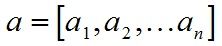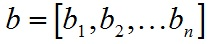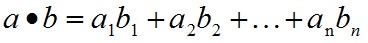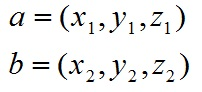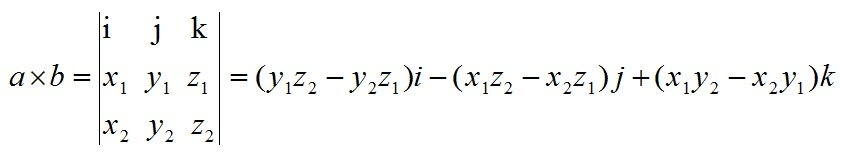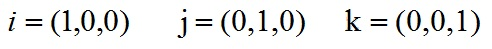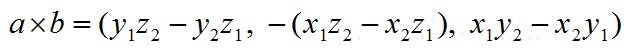From -牧野-

二、矩阵及其运算

M文件输入矩阵，M文件是一种可以在MATLAB系统中运行的文本文件，它可以分为命令式文件和函数式文件，主要使用命令式的M文件创建矩阵。

MATLAB涉及的文件类型有.m .mat .asv .fig .xml .sxl等许多种，MATLAB提供了程序设计功能，即对应以.m为扩展名的文件，简称M文件。M文件有两种形式：命令式文件（Script）和函数式文件（Function）。

$$\begin{cases} x+y+z=8\ 2x+3y-z=7\ 5x-2y+z=3 \end{cases}\ \ \ x=1\ \ \ y=3\ \ \ z=4$$

符号计算

一、符号变量与符号表达式

findsym(f,n)可以查询符号表达式使用到了哪n个符号变量，n缺省时表示查询全部。

digits(D)表示函数设置有效数字个数为D的近似解精度，
vpa(S,D)表示符号表达式S在digits(D)精度下的数值解，
subs(S,a,x)表示用x替换符号表达式S中的变量a，产生新的符号表达式，原符号表达式不变。

二、符号微积分

diff(S,v,n)表示对表达式S关于变量v求n阶导数，n省略求1阶导，v省略则对默认变量求导。

int(S,v,a,b)表示对符号表达式关于指定变量v在区间[a,b]内求定积分，若出现无穷区间情形以inf代替。
a,b两变量省略的话，求的就是不定积分。

$$\int \frac{dx}{x^2 \sqrt{1+x^2}}\ \ \ \ \ \ \ \ \ \int_{-∞}^{+∞} \frac{dx}{1+9x^2}$$

limit(S,x,a)表示计算符号表达式S在x->a时的极限。

$$\lim_{x \to 2} \frac{x^2 -4}{x-2}$$

symsum(S,v,a,b)表示对符号表达式S关于指定变量v从a到b求和。

$$\sum\limits_{k=1}^{∞}\frac{1}{k^2}$$

taylor(S,n)表示对符号函数S关于默认变量的n次麦克劳林展开式。
taylor(S,x,a,’order’,n)表示对符号函数S关于指定变量x在a点的n次泰勒展开式。

三、符号简化

factor(S)表示把表达式S分解为多个因式，各因式的系数均为有理数，若S是个整数则对S进行素数分解。

expand(S)表示把表达式S分解进行展开。

collect(S,v)表示对表达式S按照变量v的同幂项进行合并。

[R,HOW]=simple(S)表示对表达式S尝试不同算法简化，找到表达式的长度最短形式R，HOW为简化过程中的主要方法，不过现在版本已经没有了。
simplify(S)表示对表达式S利用恒等式进行化简。

[N,D]=numden(S)表示将表达式S的各元素转换为分子和分母都是整系数的最佳多项式，N表示分子D表示分母。

horner(S)表示将符号多项式S转换成嵌套形式表示，用多层括号表示。

图形可视化

一、二维图形的绘制

MATLAB作图需要先取得图形上一系列的点坐标，即横纵坐标，然后调用plot函数绘制曲线。
plot(x,y,’s’)中x是横坐标向量，y是纵坐标向量，s为选项字符串，可以控制线型和颜色。
plot(xk,yk,’s’…)可以绘制多条曲线，但要求各x横坐标向量维数相同，各y纵坐标向量维数相同。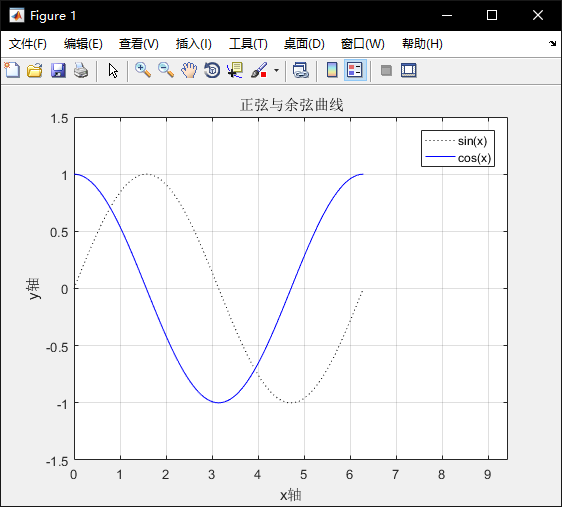subplot(m,n,p)表示将图形窗口分割为mxn个绘图区，每行n个共m行，区号按行优先编号，且选定第p个区为当前活动区。

ezplot(‘f’,[xmin,xmax,ymin,ymax])表示绘制函数f在xmin<x<xmax，ymin<y<ymax内的图形，当区间默认时为[-2pi,2pi]。
ezplot(x,y,[tmin,tmax])表示绘制参数方程x=x(t)与y=y(t)在区间tmin<t<tmax内的图形。
fplot(‘fun’,[xmin,xmax])表示绘制字符串fun所指定的函数在xmin<x<xmax内的图形，fun必须是M文件的函数名或者是独立变量x的字符串。

f1=sinx,x∈[-4pi,4pi]；f2=x^2/4-y^2/9=1,x∈[-5,5],y∈[-3,3]；f3=exp(x)+sinx,x∈[-1,1]。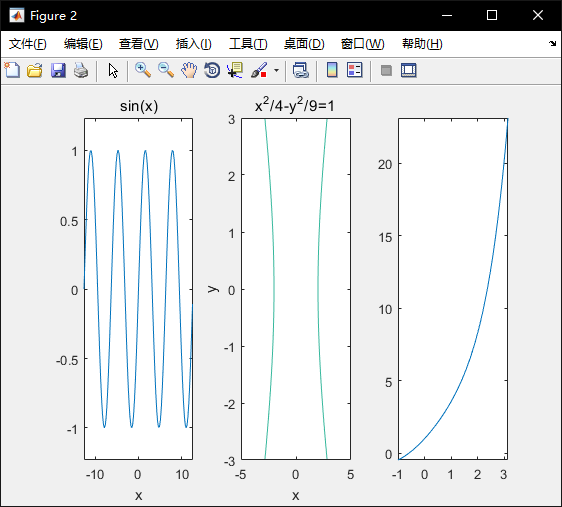二、三维图形的绘制

plot3(x,y,z)表示绘制一条三维曲线，其中x,y,z为三个相同维数的向量，函数绘出这些向量所表示的三维曲线。
plot3(X,Y,Z)绘制多条三维曲线，X,Y,Z是三个相同阶数的矩阵，绘出的是三个矩阵列向量表示的曲线。
plot3(xk,yk,zk,ck…)也可以绘制多条，c表示线型和颜色。

x=2cost,y=2sint,z=3t,t∈[0,10pi]。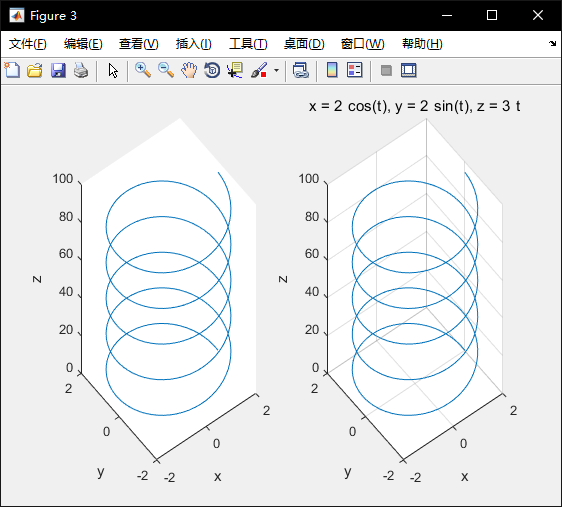[X,Y]=meshgrid(x,y)，X,Y矩阵元素分别表示为所绘曲面在XOY面投影点的x,y轴坐标值，若向量x的维数为m，向量y的维数为n，则X,Y矩阵对应维数为nxm->利用函数z=f(X,Y)，计算函数值->利用MATLAB三维曲面绘制函数，绘制三维曲面图。
mesh(X,Y,Z,C)绘制三维曲面网格图，C控制网格颜色。
surf(X,Y,Z,C)绘制三维曲面颜色填充图，C控制网格内区域颜色。

az是指，x平行于观察者身体，y垂直于观察者身体，az=0，绕z顺时针运动az为正，逆时针为负。
el是指，当观察者眼睛在xy平面上el=0，向上el为正，向下为负。需要指定点时则view([x,y,z])。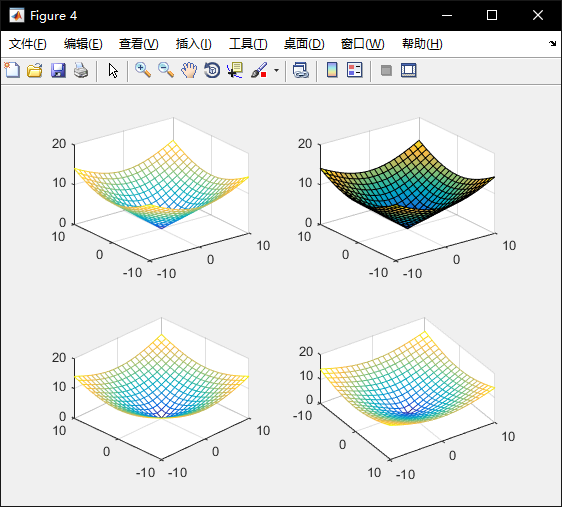ezmesh(z(x,y),[xmin,xmax,ymin,ymax])表示在x和y的范围内绘制z。
ez前缀的这几个函数用于处理符号函数，而不加的surf，mesh等用来处理数值函数。